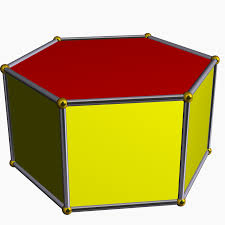# Calculate 66254

Calculate the volume and surface of a regular hexagonal prism with a height v = 2cm and a base edge a = 8cm.

V =  332.5538 cm3

### Step-by-step explanation:Did you find an error or inaccuracy? Feel free to write us. Thank you!

Tips for related online calculators
Need help calculating sum, simplifying, or multiplying fractions? Try our fraction calculator.